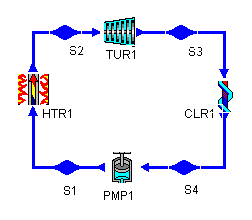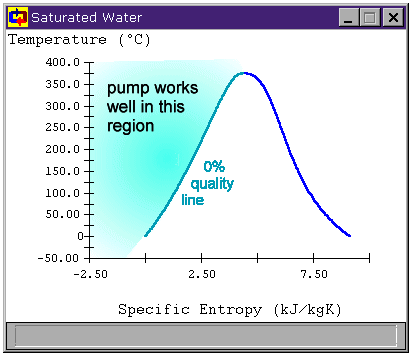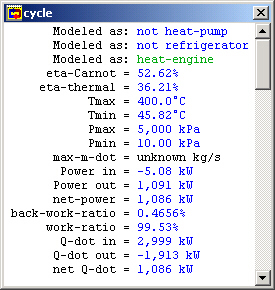# Setting Up a Rankine Cycle Using CyclePad

## Our Purpose

We would like to outline the steps involved in setting up a typical Rankine cycle, which is a very common ideal steam power cycle. Using CyclePad, setting up such a cycle is actually very simple, but it requires that we know some of the basic facts and typical assumptions that apply to the cycle. We will examine a typical Rankine cycle problem and note the assumptions necessary to find the problem's solution, many of which will not be stated explicitly in the problem.

## A typical Problem:

Let's say we want to set up a typical Rankine Cycle. A typical problem statement is:

Consider an ideal steam cycle in which steam enters the turbine at 5 MPa, 400ºC, and exits at 10 kPa. Calculate the thermal efficiency and the net work per kilogram of steam.

This may not sound like a very complete problem description, since we are given only three numbers. However, it is sufficiently described that we can solve it. Here we will detail the other properties of a Rankine cycle which allow us to complete its design.

## What it looks like:

First, an "ideal steam cycle" where we are only told of one turbine is probably a Rankine cycle. This cycle consists of a heater, a turbine, a cooler (or "condenser"), and a pump, in that order. We will talk about the properties of each component and the statepoints between them later. Right now we have enough to set up the cycle's basic layout.

The layout shown below is a clickable image. To jump to the part of this page that details the assumptions of a particular device or statepoint, just click on it.Figure 1: a Rankine cycle
Heater
Turbine Inlet
Turbine
Turbine Outlet
Cooler
Pump Inlet
Pump
Pump Outlet

## Analyzing our design:

With the design layout complete, we turn to adding the assumptions which allow CyclePad to solve the cycle. For this example, we will go around the design and add what information we know as we go, clicking on each device or statepoint to get its meter window to show up. It is particularly during this stage that our own knowledge of thermodynamics is critical to making assumptions CyclePad will use in design solution. We start at the heater.

## The Heater (HTR1)Figure 2: possible heater assumptions

We aren't explicitly told anything about the heater, but we know that a Rankine cycle has ideal components. Heaters are usually a long series of tubes through which the working fluid is forced. As it moves through the tubes, heat (from a combustion process, for instance) is applied to the outside of the tubes and the working fluid gains enthalpy. In real heaters, it takes energy to puch the fluid through all of these tubes and there is a pressure loss for the process. In an ideal heater, we assume that this pressure loss is negligible and the heater is isobaric.

(When we consider non-ideal components, we are often given a pressure loss (or a relation that allows us to compute a pressure loss) for the heater. In that case, we could enter the pressure loss as delta-P for the heater.)Figure 3: Turbine inlet meter window

## The Turbine Inlet (S2)

Looking now at the statepoint after the heater, we know both the pressure and temperature of the fluid at this statepoint from the problem statement. In addition, we are dealing with a steam cycle, so our working fluid is made of water.

We are also given the temperature and pressure at this statepoint. Entering their values, this statepoint's intensive values are completely determined.

## The Turbine (TUR1)

The next component is the turbine. While we aren't told anything explicitly about this turbine, it is ideal. In general, this means it is isentropic (zero change in entropy) and adiabatic (no heat transfer).

## The Turbine Outlet (S3)

At the outlet of the turbine, we notice that the specific entropy is already known. (It is the same as that for the statepoint before the turbine because the turbine is isentropic.) We are told in the problem that steam exits at 10 kPa, so we assume the pressure at this statepoint to have that value. Since we now know both the pressure and the entropy at this statepoint, we know all of the intensive property values here. (Once again, CyclePad has saved us much time in table lookup, especially since this statepoint would require interpolation to find.)

### Other Turbine Outlet Assumptions

We are told the turbine outlet pressure in this problem, and that is the most common case, but there are other possibilities as well. We might instead know:

• The turbine pressure ratio (PR). In this case, there would be nothing to enter at S3, and we would assume a value for PR in the turbine's meter window.
• The turbine outlet quality. Turbines can typically only handle fluid down to a certain quality; lower qualities can damage them. But we want the quality to be as low as the turbine can handle in order to extract the most energy from the working fluid. In such cases, we often assume a quality at the turbine outlet and let the state be determined by that and the outlet entropy.

## The Cooler (CLR1)Figure 4: Preparing the fluid to enter the pump

## The Pump Inlet (S4)

This is a statepoint when our own knowledge and reasoning about cycles is key to making assumptions. The only reason we have a cooler before the pump at all is because we know pumps can be damaged by non-liquids. To avoid this, the cooler must condense all of the steam leaving the turbine into a liquid before we send it to the pump. So we at least want to cool our saturated working fluid to 0% quality before sending it to the pump. Figure 4 shows the region to which we must cool the working fluid before safely using a pump.

Of course, pumps can work with compressed liquids as well, so we could cool the fluid even past saturated fluid down into the compressed liquid region. We do not do this for several reasons related to cycle efficiency.

For CyclePad, we can specify that a fluid be saturated by selecting a phase and choosing it to be saturated. Once we have told CyclePad that the phase is saturated, it adds another property to the meter window, allowing us to specify a quality. In this case, we want to assume that quality is zero, since we are forcing the fluid at S4 to be a saturated liquid.

### Other Pump Inlet Assumptions

Sometimes we can't cool the working fluid just until it is a saturated liquid. Most often this is because our cooling source is at a specified temperature and we cannot remove the working fluid from the cooler early enough. In these cases, we are usually given a temperature for this state.

## The Pump (PMP1)

We are given no explicit information about the pump, but, like the turbines, ideal pumps are adiabatic and isentropic. We assume both of those things here.

### Note

CyclePad uses the equation wpump = -vDP to calculate reversable pump work because the fluid (liquid water) is very close to incompressible. Sometimes CyclePad finds a small heat transfer for the pump and, because this heat transfer isn't quite zero, CyclePad asserts that the pump is not adiabatic or causes a contradiction when we assert that the pump is adiabatic.

Why does this happen? The reason lays in the approximation that the water in the pump is incompressible, which is very close to accurate, but the slight variation in v between the saturated liquid at the inlet and the compressed liquid at the outlet causes this small heat transfer to show up, confusing CyclePad. This is more likely at low pump inlet pressures (under one atmosphere) than at higher ones.

For our purposes, this heat transfer is not important (it is typically on the order of 0.1% of the work done by the pump), so we can just not worry about whether the pump is adiabatic if some heat transfer has already been found or the adiabatic assumption causes a contradiction.

## Finishing the problem

Our original problem was to find the thermal efficiency of this cycle. Go to the "Cycle" menu and choose "Cycle Properties". This meter window is mostly empty because CyclePad doesn't know which definitions for efficiencies to use and they are different for a heat engine (like our Rankine cycle) than they would be for a refrigeration cycle, for instance. Here we need to tell CyclePad that we are modeling a heat engine. We see that the Carnot efficiency is just over 56.6%.

However, we notice that CyclePad still does not know the many of the values in this cycle. Among the unknown values is the net work per kg of steam and the thermal efficiency, which we are asked to find in the problem statement. The reason is that CyclePad finds some whole cycle properties based on extensive values, which are not known until we assume a mass flow rate (m-dot). (In other words, CyclePad needs the whole heat transfer Q, not the specific heat transfer q).Figure 5: Whole cycle properties

So how do we find the net work per kg of steam? We do know that the only two devices that do work in this cycle are the pump and the turbine. So we could simply look at the net work per kg of steam for each of them (w in their meter windows) and add them together. This is how we would do the problem on paper, so we know it works.

But a more clever solution is just to assume a mass flow rate so that CyclePad can compute the extensive values it needs to finish the whole cycle calculations. At state S2, we can assume a mass flow rate of 1 kg/sec. Now, we see that those other properties are calculated and, since we have assumed a mass flow of 1 kg/sec, these numbers are per kg of steam.